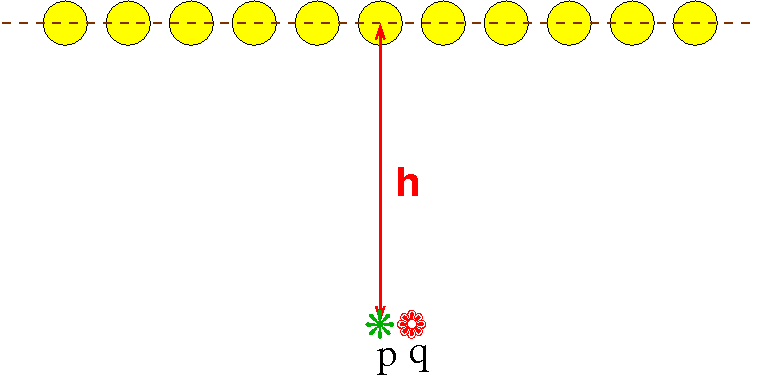# CG Homework 7, due Thu 2016-10-27 9am

1. (4 pts) Use the homogeneous matrix to project homogeneous points onto the plane x+2y+4z=5, with COP at the origin. What does the point (1,2,4,5) project to? Give the answer as a Cartesian point.

2. (4 pts) Repeat the previous question with the COP changed to (1,1,1,1).

3. (6 pts) Do exercise 5.6 on page 272 of the text,

4. (6 pts) This question will take some thinking).

Imagine that you have an infinitely large room illuminated by one infinitely long row of point lights. This figure shows a side view of the room.

The lights are h above the floor and are 1 meter from each other. Assume that the ceiling above the lights is black and that no light reflects off of anything.

An object at distance d from a light gets illuminated with a brightness $\frac{1}{d^2}$.

Each point on the floor is illuminated by all the lights, but more brightly by the closer lights.

A point p directly below a light will be a little brighter than a point q halfway between two such points. That is the problem --- we want the floor (at least the part directly below the line of lights) to be evenly lit, at least within 1%.

However, the higher the line of lights, the more evenly the floor will be lit.

Your question is to tell us what is the minimum value for h so that the line of the floor below the line of lights is evenly lit within 1%.E.g., the brightness at p is

$\sum_{i=-\infty}^{\infty} \;\;\; \frac{1}{h^2+i^2}$

(Total: 20 points.)